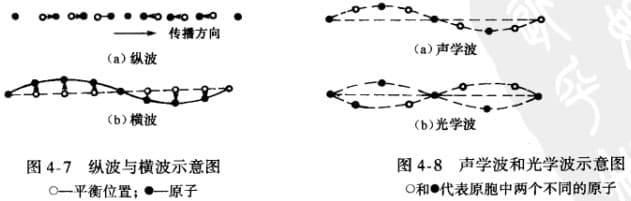# 电导的微观理论

## 欧姆定律的微分形式

$\sigma$ 是电导率，与载流子浓度、载流子的迁移率有关（在下一节推导）。

## 漂移速度和迁移率

$\mu$ 为电子的迁移率，单位为 $\mathrm{cm^2/V\cdot s}$，物理意义表示电子在电场作用下做漂移运动的难易程度。所以：

## 半导体的电导率和迁移率

1. 对于 n 型半导体：$\sigma=nq\mu_n$
2. 对于 p 型半导体：$\sigma=pq\mu_p$
3. 对于本征半导体：$\sigma_i=n_iq(\mu_n+\mu_p)$，$n=p=n_i$

# 载流子的散射

## 平均自由时间

$\tau$ 的物理意义是，所有电子从 $t=0$ 开始加速自由运动，平均来说 $t=\tau$ 时受到一次散射。

## 主要的散射机构

### 电离杂质散射1. 杂质浓度越高 $N_i$，散射几率越大；
2. 温度越高，载流子平均热运动速度越大，散射几率越小。

### 晶格振动散射

1. 声学波（整体）
1. 横波
2. 纵波
2. 光学波（相对）
1. 横波
2. 纵波#### 声子

$\vec{q}=2\pi/\lambda$ 表示格波的波矢（波长及其传播方向）,其能量是量子化的。